Smartick is a fun way to learn math!Oct28

# Calculating the Area of Polygons

In today’s post, I am going to show you some exercises we have recently incorporated into Smartick for calculating the area of polygons.

For some time we have had exercises to practice the area of figures with small squares.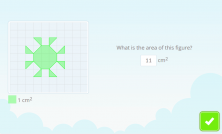The way to calculate the area of these figures is very simple because all you have to do is count the number of colored squares on the grid.

You can try it on your own by counting the squares that make up the figure in the image. Be careful when counting the triangles!

With the new exercises you can practice calculating the area of different types of polygons:

• squares
• rectangles
• right triangles

We are going to see some in these exercises.

### Calculating the area of a polygon on a grid with the measurement of the area of a square

In these exercises, a polygon is drawn on the grid and a legend is given which shows the area measurement for a square.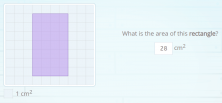To calculate the area of this rectangle, you just have to count the number of colored squares.

In this case, there are 28, and since each one of them has an area of 1cm², you can easily calculate the area of the rectangle as 28cm².

### Calculating the area of a polygon on a grid with the measurements of each side of the polygon

In these exercises, the polygon is also drawn on the grid, but in this case, the legend gives us the measurements for each side of the polygon.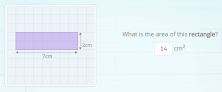To calculate the area of this rectangle, after practicing exercises of the previous type, you will have noticed that you simply multiply the measurements of each side.

In this case, the measurements are 7cm and 2cm and since 7cm x 2cm = 14cm², the area of the rectangle is 14cm².

### Find out the formula for a polygon with the measurements of each side

In these exercises, the polygon is shown without the grid and with a legend showing the measurements of each side.

There are 4 possible formulas to use when calculating the area of a polygon and it is up to you to choose the correct one.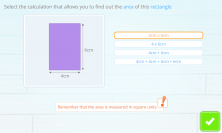Can you rule out the incorrect formulas here?

• Since 6 x 4cm = 24cm, and “cm” is a measurement of length, 24cm cannot be the area measurement of a rectangle because the area is measured in square units.
• On the other hand, 6cm + 4cm = 10cm, since this isn’t measured in square units either, this is not the area measurement of a rectangle.
• And finally, 6cm + 6cm + 4cm + 4cm = 20cm, so it cannot be the area measurement of a rectangle either.

The correct formula to calculate the area of a rectangle, as you have seen the earlier exercise, it is the product of the measurements of the sides: 6cm x 4cm = 24cm².

In later, more difficult exercises, you’ll be asked to find out the formula without the polygon being drawn.

### Draw a polygon with a specific area

These are my favorite!

In them, you are asked to draw a polygon with a specific area using the points given connected by lines.

The points and the lines are interactive and allow you to create polygons. It’s so fun to move them and create figures!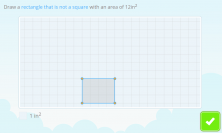In this case, to get a rectangle with an area of 12in², you can draw one that has a base of 6in and a height of 2in.

Its area will be 6in x 2in = 12in², just the area requested!

And now these new exercises calculating areas have been incorporated into Smartick’s daily sessions. What do you think? Leave us your feedback!